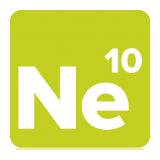Project Ne10 An open, optimized software library for the ARM architecture.
NE10_fft_bfly.h File Reference
`#include "NE10_types.h"`
`#include "NE10_fft_cplx_ops.h"`
`#include "NE10_fft_common_varibles.h"`

Go to the source code of this file.

## Macros

#define NE10_FFT_R2C_4R_RCR(OUT, IN)

#define NE10_FFT_C2R_RCR_4R(OUT, IN)

#define NE10_FFT_R2C_4R_CC(OUT, IN)

#define NE10_FFT_C2R_CC_4R(OUT, IN)

#define NE10_FFT_R2C_CC_CC(OUT, IN)

#define NE10_FFT_C2R_CC_CC(OUT, IN)

## Macro Definition Documentation

 #define NE10_FFT_C2R_CC_4R ( OUT, IN )
Value:
do { \
ne10_float32_t SCRATCH ; \
OUT = ( IN + IN); \
OUT = (-IN + IN); \
OUT = OUT + OUT; \
OUT = OUT + OUT; \
SCRATCH = (IN - IN); \
SCRATCH = (IN + IN); \
SCRATCH = (SCRATCH + SCRATCH); \
SCRATCH = (SCRATCH - SCRATCH); \
OUT = SCRATCH / TW_81N_F32; \
OUT = SCRATCH / TW_81_F32; \
} while (0)
float ne10_float32_t
Definition: NE10_types.h:80

Definition at line 87 of file NE10_fft_bfly.h.

 #define NE10_FFT_C2R_CC_CC ( OUT, IN )
Value:
do { \
ne10_fft_cpx_float32_t SCRATCH; \
SCRATCH.r = (IN.r + IN.r); \
SCRATCH.r = (IN.r - IN.r); \
SCRATCH.i = (IN.i + IN.i); \
SCRATCH.i = (IN.i - IN.i); \
SCRATCH.r = (IN.r + IN.r); \
SCRATCH.i = (IN.r - IN.r); \
SCRATCH.r = (IN.i + IN.i) * -1.0f; \
SCRATCH.i = (IN.i - IN.i); \
OUT.r = (SCRATCH.r + SCRATCH.r); \
OUT.r = (SCRATCH.r - SCRATCH.r); \
OUT.i = (SCRATCH.i + SCRATCH.i); \
OUT.i = (SCRATCH.i - SCRATCH.i); \
OUT.r = (SCRATCH.r + SCRATCH.r); \
OUT.r = (SCRATCH.r - SCRATCH.r); \
OUT.i = (SCRATCH.i + SCRATCH.i); \
OUT.i = (SCRATCH.i - SCRATCH.i); \
} while (0)

Definition at line 129 of file NE10_fft_bfly.h.

 #define NE10_FFT_C2R_RCR_4R ( OUT, IN )
Value:
do { \
ne10_float32_t SCRATCH ; \
SCRATCH =(IN + IN); \
SCRATCH =(IN - IN); \
SCRATCH = IN + IN; \
SCRATCH = IN + IN; \
OUT = SCRATCH + SCRATCH; \
OUT = SCRATCH - SCRATCH; \
OUT = SCRATCH - SCRATCH; \
OUT = SCRATCH + SCRATCH; \
} while (0)
float ne10_float32_t
Definition: NE10_types.h:80

Definition at line 56 of file NE10_fft_bfly.h.

 #define NE10_FFT_R2C_4R_CC ( OUT, IN )
Value:
do { \
ne10_float32_t SCRATCH ; \
ne10_float32_t TMP ; \
SCRATCH = (IN - IN) * TW_81N_F32; \
SCRATCH = (IN + IN) * TW_81N_F32; \
OUT = IN + SCRATCH; \
OUT = IN - SCRATCH; \
OUT = SCRATCH - IN; \
OUT = SCRATCH + IN; \
} while (0)
float ne10_float32_t
Definition: NE10_types.h:80

Definition at line 72 of file NE10_fft_bfly.h.

 #define NE10_FFT_R2C_4R_RCR ( OUT, IN )
Value:
do { \
ne10_float32_t SCRATCH ; \
SCRATCH = IN + IN; \
SCRATCH = IN + IN; \
OUT = SCRATCH + SCRATCH; \
OUT = IN - IN; \
OUT = IN - IN; \
OUT = SCRATCH - SCRATCH; \
} while (0)
float ne10_float32_t
Definition: NE10_types.h:80

Definition at line 42 of file NE10_fft_bfly.h.

 #define NE10_FFT_R2C_CC_CC ( OUT, IN )
Value:
do { \
ne10_fft_cpx_float32_t TMP; \
ne10_float32_t TMP_SWAP; \
NE10_CPX_SUB (TMP, IN, IN); \
NE10_CPX_SUB (TMP, IN, IN); \
TMP_SWAP = TMP.i; \
TMP.i = - TMP.r; \
TMP.r = TMP_SWAP; \
OUT.r = TMP.r + TMP.r; \
OUT.i = TMP.i + TMP.i; \
OUT.r = TMP.r - TMP.r; \
OUT.i = -(TMP.i - TMP.i); \
OUT.r = TMP.r + TMP.r; \
OUT.i = TMP.i + TMP.i; \
OUT.r = TMP.r - TMP.r; \
OUT.i = -(TMP.i - TMP.i); \
} while (0)
float ne10_float32_t
Definition: NE10_types.h:80